# Map Scale Class 8 Geography Notes Maharashtra Board

## Map Scale Class 8 Geography Notes Maharashtra State Board

The painter first takes an estimated scale of the scene in front of him with a pencil. After that, he sketches on the paper. The painter does this so that the picture is drawn to scale.In the preparation of maps, the picture is first surveyed. At that time after special observation, a scale is fixed. Using this scale, an outline map of the whole earth or a part of it is prepared.We obtain information about the earth or some specific part of it from a globe or a map. While preparing a map, to get the actual ground distances onto paper, some conversions have to be made using Geometry and Mathematics. The use of a map scale is useful for this conversion. Let us get acquainted with this aspect of maps.In a map scale, it is essential to correlate the distance between two points on a map and the actual distance between these two points on the ground. The ratio of the map distance to the ground distance is the map scale.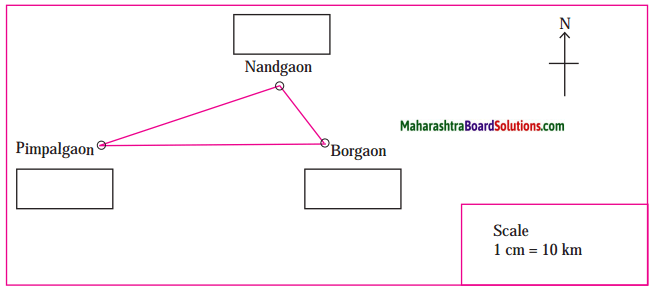To draw a map true to scale it is essential to know the ground distances. These distances are measured during surveying. While preparing a map, based on the distances a suitable ratio is selected. After the preparation of the map, it is necessary to mention the map scale. This facilitates map reading and helps in understanding the distances on the ground. There are three ways of expressing the scale on a map.

• Verbal Scale
• Numerical Scale
• Linear Scale/Graphical Scale

In the figure, a map is given. All the above methods of map scale are shown on the map. Carefully note the different ways of writing the scale.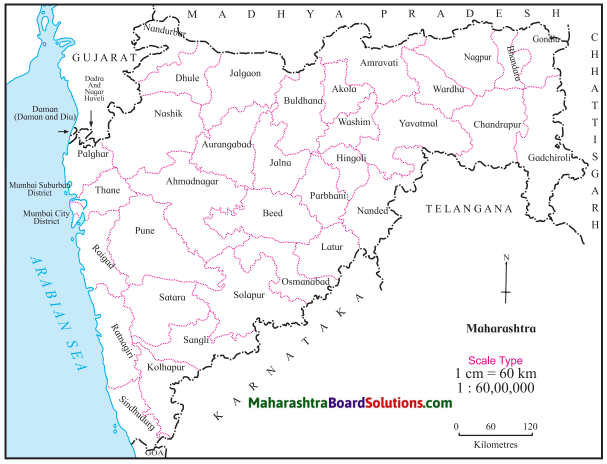1. Verbal Scale:
A verbal scale is one in which distances are expressed with the use of words indicating measurement e.g. if the scale is 1 cm = 60 km then cm is the unit of distance on the map and km, is the unit of distance on the ground.

2. Numerical Scale:
The scale is expressed as a ratio. E.g. in 1:60,00,000. 1 represents the distance on the map, and 60,00,000 represents the distance on the ground. This is 60,00,000 times the map distance. It is also known as a representative fraction. In this scale, only numbers are used. This scale is also written as 1:60,00,000. In this method, there are no words used indicating measurement. The same unit used for the figures on the left-hand side is also used for the figures on the right-hand side.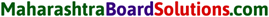3. Linear Scale:
This scale is shown graphically on a map. The distance between any two points on a map is measured with the help of a linear/graphical scale e.g.If a ruler is not available then a compass, a blade of grass, or for curves a thread can be used for measuring the actual distances between places on a map. You will understand this with the help of the next exercise.

The Importance of Graphical Scale:
Very often it is necessary to reduce or enlarge the original map. During that process the scale of the map changes. There are many methods of reducing or enlarging maps. One of them is by taking a photocopy of the original map and reducing or enlarging it. When the map is reduced or enlarged by this method the verbal and the numerical scale on the original map does not change. But if a graphical scale is drawn on the original map, then the scale changes as per the changing size of the map. Although the font size of the vebal and numerical scale changes the values do not change. Hence graphical scales are drawn in atlas and wall maps.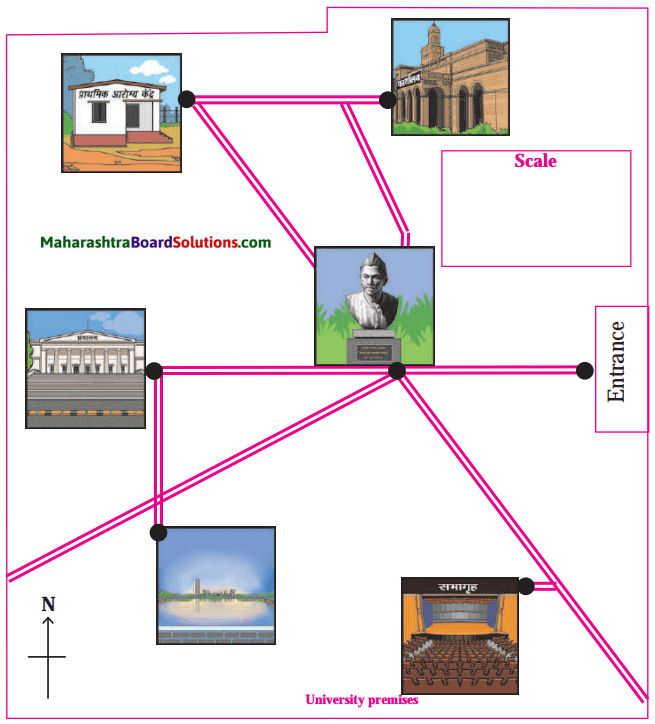Large Scale and Small Scale Maps:
When a small area on the ground is shown covering a large area on the map, it is a large-scale map. Maps of a city, village, agricultural fields, etc. are all examples of large-scale maps. Generally maps with a scale of less than 1:10,000 are known as large-scale maps.In mathematics when we compare two fractions then the fraction with the smaller number in the denomination has a higher value. Map scales are not in fractions but they are ratios. The same principle of fractions is used while comparing ratios. Hence a numerical scale of 1:10,000 is called a large scale and a numerical scale of 1:50,000 is a small scale.When a part of the ground covers a small area on the map, they are small-scale maps. That means when a small scale is used to show information about a large part of the earth, these are small-scale maps. Maps in an atlas and world maps are examples of small-scale maps. Most of the maps in an atlas are examples of small-scale maps.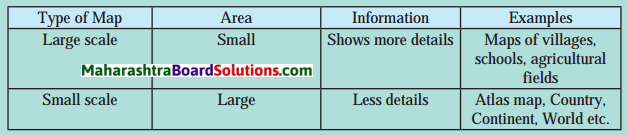On the map, the left side of the scale shows the distance on the map and the right side shows the actual distance on the ground.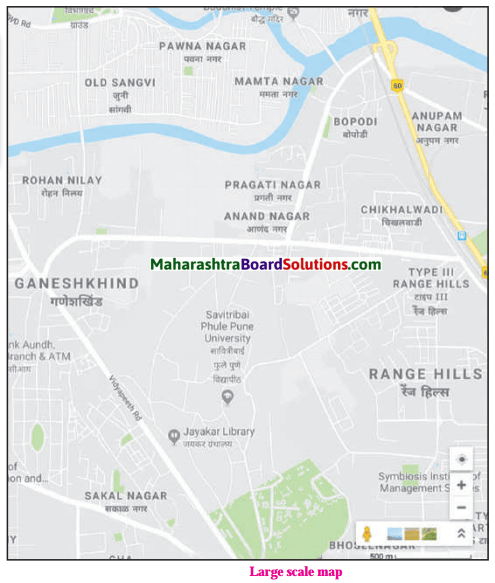Different countries use different units of measurement. This could become a limitation while reading a map. Hence it is necessary to have a numerical scale on maps. The numerical scale is global.

Students often rely on the comprehensive Maharashtra State Board Class 8 Geography Notes Map Scale to excel in their classes.